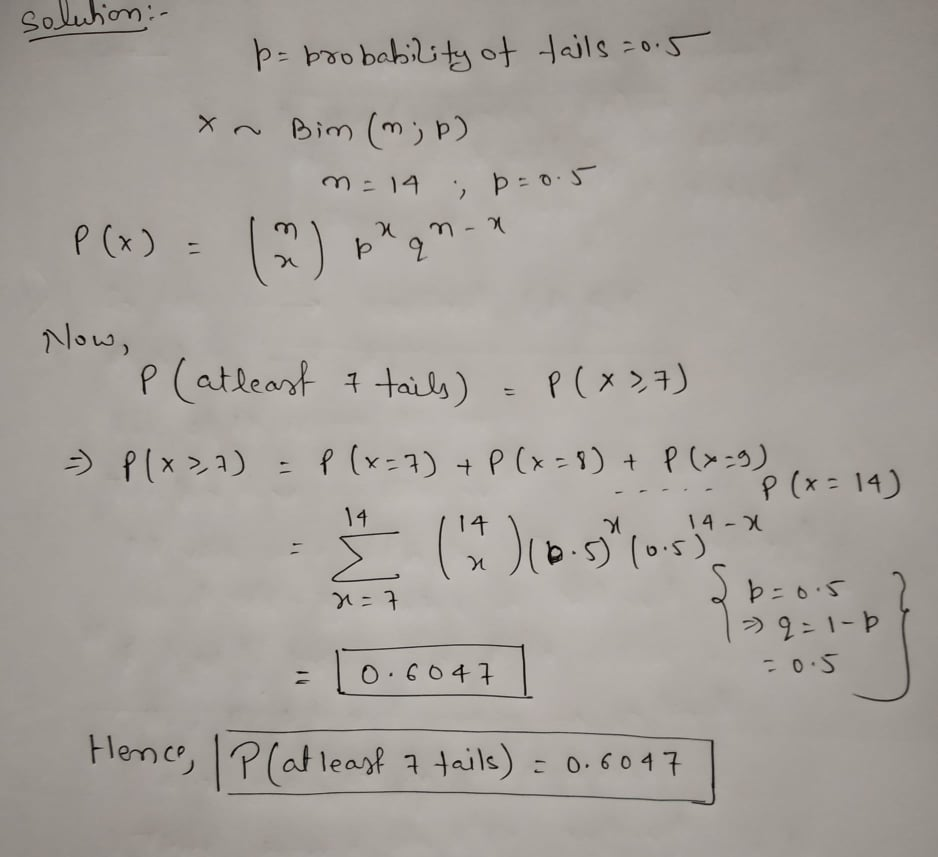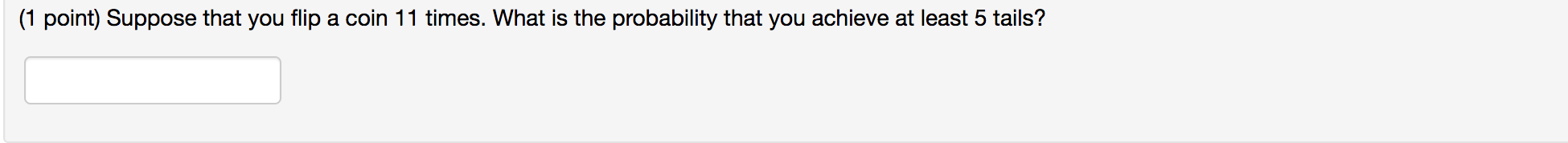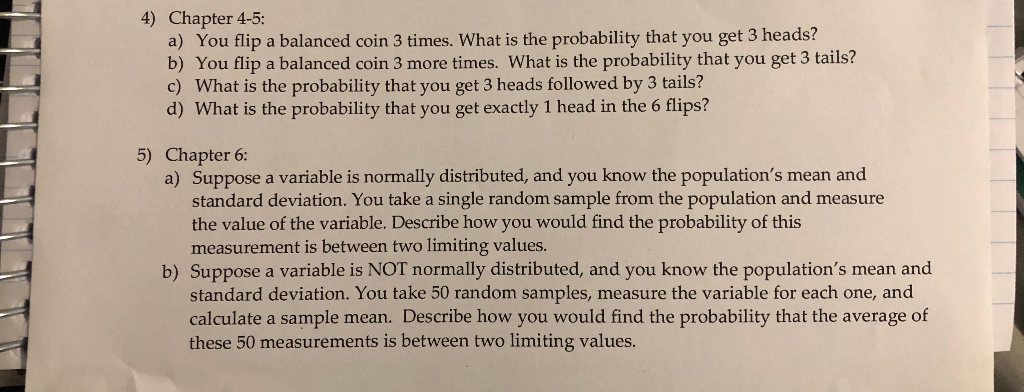Question

# Suppose that you flip a coin 14 times. What is the probability that you achieve at...

Suppose that you flip a coin 14 times. What is the probability that you achieve at least 7 tails?#### Earn Coins

Coins can be redeemed for fabulous gifts.

Similar Homework Help Questions
• ### Suppose that you flip a coin 15 times. What is the probability that you achieve at least 6 tails?

Suppose that you flip a coin 15 times. What is the probability that you achieve at least 6 tails?

• ### (1 point) Suppose that you flip a coin 11 times. What is the probability that you...(1 point) Suppose that you flip a coin 11 times. What is the probability that you achieve at least 5 tails?

• ### Coin Flips:  If you flip a fair coin 5 times, what is the probability of each of...

Coin Flips:  If you flip a fair coin 5 times, what is the probability of each of the following? (please round all answers to 4 decimal places) a) getting all tails? b) getting all heads?

• ### If you flip a fair coin six times, what is the probability of having more heads...

If you flip a fair coin six times, what is the probability of having more heads than tails?

• ### Suppose that I flip a fair coin 21 times. What is the probability that it will...

Suppose that I flip a fair coin 21 times. What is the probability that it will land on heads exactly 13 times?

• ### Suppose that I flip a fair coin 36 times. What is the probability that it will...

Suppose that I flip a fair coin 36 times. What is the probability that it will land on heads exactly 23 times?

• ### Suppose that I flip a fair coin 21 times. What is the probability that it will...

Suppose that I flip a fair coin 21 times. What is the probability that it will land on heads exactly 12 times? Round to 4 decimal places.

• ### Suppose that I flip a fair coin 34 times. What is the probability that it will...

Suppose that I flip a fair coin 34 times. What is the probability that it will land on heads exactly 19 times? Make sure your answer is between 0 and 1.

• ### PLEASE ANSWER QUESTION # 5 a and b !!!!!!!!!! 4) Chapter 4-5: a) You flip a balanced coin 3 times. What is the probabil...PLEASE ANSWER QUESTION # 5 a and b !!!!!!!!!! 4) Chapter 4-5: a) You flip a balanced coin 3 times. What is the probability that you get 3 heads? b) You flip a balanced coin 3 more times. What is the probability that you get 3 tails? c) What is the probability that you get 3 heads followed by 3 tails? d) What is the probability that you get exactly 1 head in the 6 flips? 5) Chapter 6: a)...

• ### We flip a fair coin 10 times. What is the probability that there are at least...

We flip a fair coin 10 times. What is the probability that there are at least 4 heads out of the 10 flips?